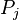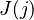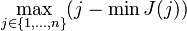# Cognitive load when learning mathematics

Jump to: navigation, search

This page lists some math-specific stuff relating to cognitive load.

Some things that increase strain on working memory:

• If a proof has steps$P_1, P_2, P_3, \ldots, P_n$ the proof is much easier to follow when the justifications for a step$P_j$ are close to$P_j$. When much earlier stages of the proof are required to understand the current step, the reader has to go back to remember what was done early on. If$J(j)$ is the set of justification numbers used on step$P_j$ (e.g. maybe$J(5) = \{1,3\}$), then the quantity of interest is something like$\max_{j\in \{1,\ldots,n\}}(j - \min J(j))$, which is the most number of steps the proof stretches backwards (or maybe it's the average of this quantity, or the average length of "stretching back"). Kind of related to space requirements for an algorithm and things like peephole optimization.
• Level of nesting: nested sums, nested products, nested quantifiers, nested loops, combinations of nesting. Ideas like common knowledge involve "indefinite nesting".
• Number of variables in use. As a special case, the number of indices in use.
• The number of new definitions/ideas introduced before getting to the "point" of the discussion.
• Numerical references, like "Lemma 5.8.9" and "Equation (63)". This is similar to the justifications one for proofs, but it also involves going outside the proof to look up statements.